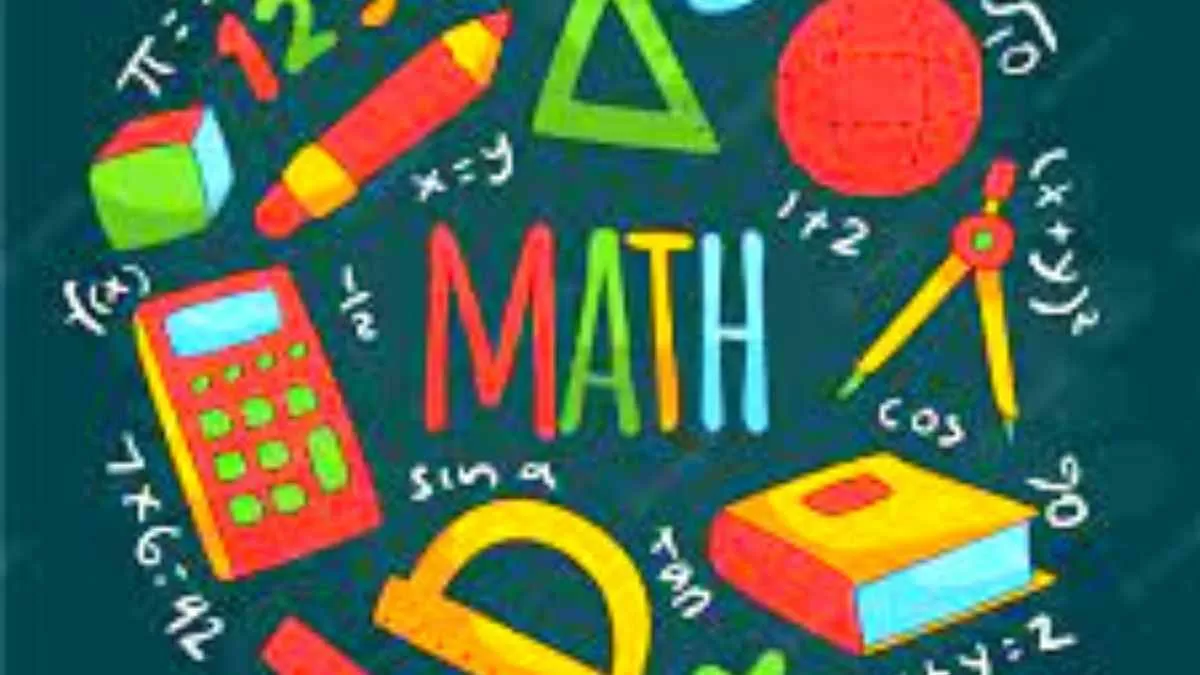# Math riddles with answers: Math is no longer boring! Don’t believe us? Here are some math riddles as evidence!

Poor math is often blamed for being uninteresting, and a headache for students. Math decided to enter the scene in a different way.Math is a great way to tease your brain a bit. Yes, we agree that the subject may have bothered you a lot during your school days, but it is not the subject that haunts you; it is the pressure to cope well with it. That is when you need to change the way you look at the subject.

Today, we bring forward some math riddles that might change your perception of the subject.

Here you go!

Math riddle 1:

Make use of only the plus sign (+) in 88888888 to get 1,000 as the answer.

Math riddle 2:

Math riddle 3:

How many 9's are present between 1 and 100?

Math riddle 1:

Make use of only the plus sign (+) in 88888888 to get 1,000 as the answer.

888 + 88 + 8 + 8 + 8 = 1,000.

Math riddle 2:

This holds on a clock.

Math riddle 3:

How many 9's are present between 1 and 100?